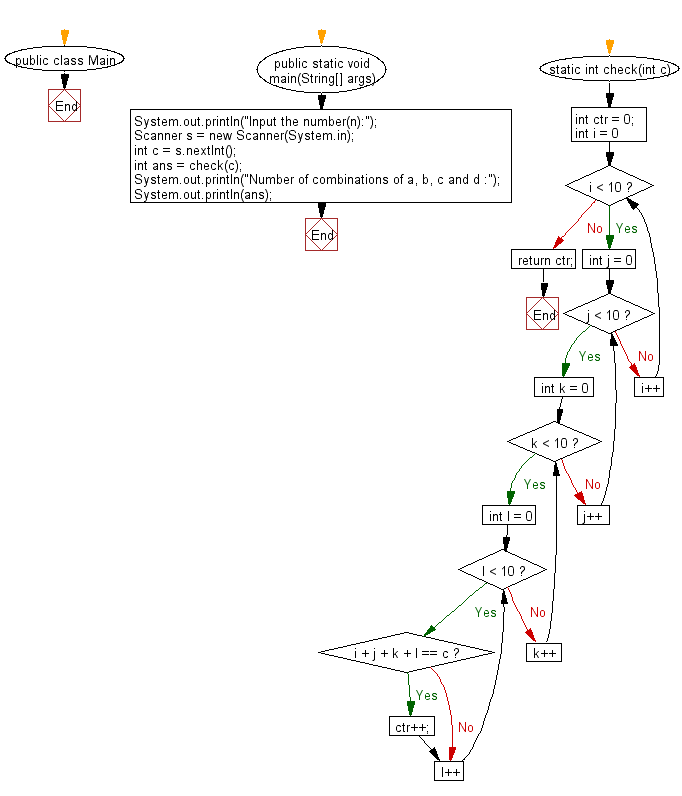# Java Exercises: Reads an integer n and find the number of combinations of a,b,c and d will be equal to n

## Java Basic: Exercise-216 with Solution

Write a Java program which reads an integer n and find the number of combinations of a,b,c and d (0 ≤ a,b,c,d ≤ 9) where (a + b + c + d) will be equal to n.

Input:

n (1 ≤ n ≤ 50).

Sample Solution:

Java Code:

`````` import java.util.Scanner;
public class Main {

public static void main(String[] args) {
System.out.println("Input the number(n):");
Scanner s = new Scanner(System.in);
int c = s.nextInt();
int ans = check(c);
System.out.println("Number of combinations of a, b, c and d :");
System.out.println(ans);
}
static int check(int c) {
int ctr = 0;
for(int i = 0; i < 10; i++) {
for(int j = 0; j < 10; j++) {
for(int k = 0; k < 10; k++) {
for(int l = 0; l < 10; l++) {
if(i + j + k + l == c) {
ctr++;
}
}
}
}
}
return ctr;
}
}
```
```

Sample Output:

```Input the number(n):
5
Number of combinations of a, b, c and d :
56
```

Flowchart:Java Code Editor:

What is the difficulty level of this exercise?

﻿

## Java: Tips of the Day

Parsing dates:

```import java.io.*;
import java.util.*;
import java.text.*;

String s = "2001/09/23 14:39";

SimpleDateFormat formatter = new SimpleDateFormat ("yyyy/MM/dd H:mm");
Date d = formatter.parse(s, new ParsePosition(0));
```